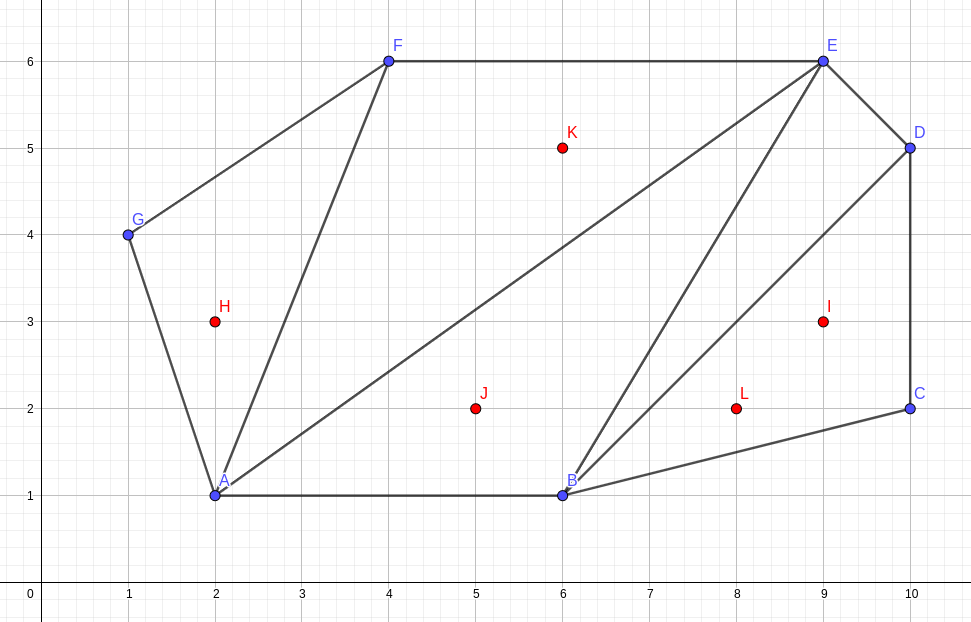O - Polygon

Languages: C, C++, Java, Python, ... (details)

You are given a convex polygon with $n$ vertices (no three of them lie on the same line) and a triangulation for that polygon. You should answer $q$ queries of the type $x_1, y_1, x_2, y_2$. For each query you should output the minimum number of diagonals you need to cross when walking from point ($x_1, y_1$) to point ($x_2, y_2$) without stepping out of the polygon.Image is related to sample input
1.  If we are at point $H$ inside $\triangle AFG$  and want to get to point $I$ in $\triangle BCD$, best route implies crossing all 4 given diagonals ($\overline{AF}$, $\overline{AE}$, $\overline{BE}$, $\overline{BD}$).
2.  If we are at point $J$ inside $\triangle ABE$  and want to get to point $K$ in $\triangle AEF$, since points are in adjacent triangles, it is sufficient to cross the diagonal $\overline{AE}$.
3.  If we are at point $L$ inside  $\triangle BCD$ and want to get to point $I$ in $\triangle BCD$, since both points are inside the same triangle, the amount of diagonals to cross is 0.

Input

First line of input contains an integer $n$ ($3 \leq n \leq 10^5$) describing the number of vertices of the polygon. $N$ lines follow, the $i$-th of them with two integers $x_i, y_i$ ($-10^9 \leq x_i, y_i \leq 10^9$), the coordinates of the $i$-th vertex of the polygon, given in counter-clockwise order.

Next $n-3$ lines describes the triangulation, giving the list of diagonals that make up the triangulation. Each of those lines contains two integers $a_i, b_i$ ($1 \leq a_i, b_i \leq n$), indicating there is a diagonal from the $a_i$-th vertex to the $b_i$-th vertex, according to the order in which vertices were given in the input. It is guaranteed that the given list of diagonals make up a valid triangulation for the polygon.
Next line gives you the integer $q$ ($1 \leq q \leq 10^5$), the number of queries to process. And the final $q$ lines contain four integers $x_1, y_1, x_2, y_2$ describing the query. It is guaranteed that both points are inside the polygon and do not lie on a given diagonal nor on a side of the polygon.

Output

For each query output a line with answer for that query.

Sample test(s)

Input
7 2 1 6 1 10 2 10 5 9 6 4 6 1 4 1 6 1 5 2 4 2 5 3 2 3 9 3 5 2 6 5 8 2 9 3
Output
4 1 0
Input
4 100 100 200 100 200 200 100 200 1 3 3 150 140 150 160 190 140 190 160 110 140 110 160
Output
1 0 0